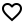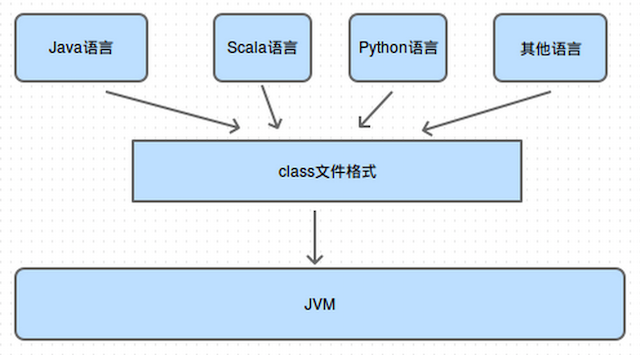## 公共技术点之 Java 反射 Reflection项目：，分析者：Mr.Simple，校对者：Trinea

### 1. 了解 Java 中的反射

#### 1.1 什么是 Java 的反射

Java 反射是可以让我们在运行时获取类的函数、属性、父类、接口等 Class 内部信息的机制。通过反射还可以让我们在运行期实例化对象，调用方法，通过调用 get/set 方法获取变量的值，即使方法或属性是私有的的也可以通过反射的形式调用，这种“看透 class”的能力被称为内省，这种能力在框架开发中尤为重要。 有些情况下，我们要使用的类在运行时才会确定，这个时候我们不能在编译期就使用它，因此只能通过反射的形式来使用在运行时才存在的类(该类符合某种特定的规范，例如 JDBC)，这是反射用得比较多的场景。

#### 1.2 Class 类### 2. 反射 Class 以及构造对象

#### 2.1 获取 Class 对象

``````Class<?> myObjectClass = MyObject.class;
``````

``````Student me = new Student("mr.simple");
Class<?> clazz = me.getClass();
``````

``````Class<?> myObjectClass = Class.forName("com.simple.User");
``````

``````// 加载指定的 Class 对象，参数 1 为要加载的类的完整路径，例如"com.simple.Student". ( 常用方式 )
public static Class<?> forName (String className)

// 加载指定的 Class 对象，参数 1 为要加载的类的完整路径，例如"com.simple.Student";
// 参数 2 为是否要初始化该 Class 对象，参数 3 为指定加载该类的 ClassLoader.
``````

### 2.2 通过 Class 对象构造目标类型的对象

``````    private static void classForName() {
try {
// 获取 Class 对象
// 通过 Class 对象获取 Constructor，Student 的构造函数有一个字符串参数
// 因此这里需要传递参数的类型 ( Student 类见后面的代码 )
Constructor<?> constructor = clz.getConstructor(String.class);
// 通过 Constructor 来创建 Student 对象
Object obj = constructor.newInstance("mr.simple");
System.out.println(" obj :  " + obj.toString());
} catch (Exception e) {
e.printStackTrace();
}
}
``````

``````// 获取一个公有的构造函数，参数为可变参数，如果构造函数有参数，那么需要将参数的类型传递给 getConstructor 方法
public Constructor<T> getConstructor (Class...<?> parameterTypes)
// 获取目标类所有的公有构造函数
public Constructor[]<?> getConstructors ()
``````

``````   Constructor<?> constructor = clz.getConstructor(String.class);
// 设置 Constructor 的 Accessible
constructor.setAccessible(true);

// 设置 Methohd 的 Accessible
Method learnMethod = Student.class.getMethod("learn"， String.class);
learnMethod.setAccessible(true);
``````

Person.java

``````public class Person {
String mName;

public Person(String aName) {
mName = aName;
}

private void sayHello(String friendName) {
System.out.println(mName + " say hello to " + friendName);
}

protected void showMyName() {
System.out.println("My name is " + mName);
}

public void breathe() {
System.out.println(" take breathe ");
}
}
``````

Student.java

``````public class Student extends Person implements Examination {
// 年级

public Student(String aName) {
super(aName);
}

public Student(int grade, String aName) {
super(aName);
}

private void learn(String course) {
System.out.println(mName + " learn " + course);
}

public void takeAnExamination() {
System.out.println(" takeAnExamination ");
}

public String toString() {
return " Student :  " + mName;
}
``````

Breathe.java

``````// 呼吸接口
public interface Breathe {
public void breathe();
}
``````

Examination.java

``````// 考试接口
public interface Examination {
public void takeAnExamination();
}
``````

### 3 反射获取类中函数

#### 3.1 获取当前类中定义的方法

`````` private static void showDeclaredMethods() {
Student student = new Student("mr.simple");
Method[] methods = student.getClass().getDeclaredMethods();
for (Method method : methods) {
System.out.println("declared method name : " + method.getName());
}

try {
Method learnMethod = student.getClass().getDeclaredMethod("learn", String.class);
// 获取方法的参数类型列表
Class<?>[] paramClasses = learnMethod.getParameterTypes() ;
for (Class<?> class1 : paramClasses) {
System.out.println("learn 方法的参数类型 : " + class1.getName());
}
// 是否是 private 函数，属性是否是 private 也可以使用这种方式判断
System.out.println(learnMethod.getName() + " is private "
+ Modifier.isPrivate(learnMethod.getModifiers()));
learnMethod.invoke(student, "java ---> ");
} catch (Exception e) {
e.printStackTrace();
}
}
``````

#### 3.2 获取当前类、父类中定义的公有方法

``````    private static void showMethods() {
Student student = new Student("mr.simple");
// 获取所有方法
Method[] methods = student.getClass().getMethods();
for (Method method : methods) {
System.out.println("method name : " + method.getName());
}

try {
// 通过 getMethod 只能获取公有方法，如果获取私有方法则会抛出异常，比如这里就会抛异常
Method learnMethod = student.getClass().getMethod("learn", String.class);
// 是否是 private 函数，属性是否是 private 也可以使用这种方式判断
System.out.println(learnMethod.getName() + " is private " + Modifier.isPrivate(learnMethod.getModifiers()));
// 调用 learn 函数
learnMethod.invoke(student, "java");
} catch (Exception e) {
e.printStackTrace();
}
}
``````

``````// 获取 Class 对象中指定函数名和参数的函数，参数一为函数名，参数 2 为参数类型列表
public Method getDeclaredMethod (String name, Class...<?> parameterTypes)

// 获取该 Class 对象中的所有函数( 不包含从父类继承的函数 )
public Method[] getDeclaredMethods ()

// 获取指定的 Class 对象中的**公有**函数，参数一为函数名，参数 2 为参数类型列表
public Method getMethod (String name, Class...<?> parameterTypes)

// 获取该 Class 对象中的所有**公有**函数 ( 包含从父类和接口类集成下来的函数 )
public Method[] getMethods ()
``````

### 4 反射获取类中的属性

#### 4.1 获取当前类中定义的属性

``````    private static void showDeclaredFields() {
Student student = new Student("mr.simple");
// 获取当前类和父类的所有公有属性
Field[] publicFields = student.getClass().getDeclaredFields();
for (Field field : publicFields) {
System.out.println("declared field name : " + field.getName());
}

try {
// 获取当前类和父类的某个公有属性
// 获取属性值
// 设置属性值
} catch (Exception e) {
e.printStackTrace();
}
}
``````

#### 4.2 获取当前类、父类中定义的公有属性

``````    private static void showFields() {
Student student = new Student("mr.simple");
// 获取当前类和父类的所有公有属性
Field[] publicFields = student.getClass().getFields();
for (Field field : publicFields) {
System.out.println("field name : " + field.getName());
}

try {
// 获取当前类和父类的某个公有属性
Field ageField = student.getClass().getField("mAge");
System.out.println(" age is : " + ageField.getInt(student));
} catch (Exception e) {
e.printStackTrace();
}
}
``````

``````// 获取 Class 对象中指定属性名的属性，参数一为属性名
public Method getDeclaredField (String name)

// 获取该 Class 对象中的所有属性( 不包含从父类继承的属性 )
public Method[] getDeclaredFields ()

// 获取指定的 Class 对象中的**公有**属性，参数一为属性名
public Method getField (String name)

// 获取该 Class 对象中的所有**公有**属性 ( 包含从父类和接口类集成下来的公有属性 )
public Method[] getFields ()
``````

### 5 反射获取父类与接口

#### 5.1 获取父类

``````    Student student = new Student("mr.simple");
Class<?> superClass = student.getClass().getSuperclass();
while (superClass != null) {
System.out.println("Student's super class is : " + superClass.getName());
// 再获取父类的上一层父类，直到最后的 Object 类，Object 的父类为 null
superClass = superClass.getSuperclass();
}
``````

#### 5.2 获取接口

``````    private static void showInterfaces() {
Student student = new Student("mr.simple");
Class<?>[] interfaceses = student.getClass().getInterfaces();
for (Class<?> class1 : interfaceses) {
System.out.println("Student's interface is : " + class1.getName());
}
}
``````

### 6 获取注解信息

``````    @Target({
ElementType.METHOD, ElementType.FIELD, ElementType.TYPE
})
@Retention(RetentionPolicy.RUNTIME)
static @interface Test {

}
``````

``````@Test(tag = "Student class Test Annoatation")
public class Student extends Person implements Examination {
// 年级
@Test(tag = "mGrade Test Annotation ")

// ......
}
``````

``````    private static void getAnnotationInfos() {
Student student = new Student("mr.simple");
Test classTest = student.getClass().getAnnotation(Test.class);
System.out.println("class Annotatation tag = " + classTest.tag());

Field field = null;
try {
Test testAnnotation = field.getAnnotation(Test.class);
System.out.println("属性的 Test 注解 tag : " + testAnnotation.tag());
} catch (Exception e) {
e.printStackTrace();
}
}
``````

``````class Annotatation tag = Student class Test Annoatation

``````

``````// 获取指定类型的注解
public <A extends Annotation> A getAnnotation(Class<A> annotationClass) ;
// 获取 Class 对象中的所有注解
public Annotation[] getAnnotations() ;
``````

## 杂谈

Apps